|

# 洁琅新风,乘势而上

2017年8月31日，第14届中国国际新风系统与空气净化产业博览会暨高峰论坛（简称净博会）在北京中国国际展览中心（静安庄）拉开帷幕，会期时间为3天。

净博会作为亚洲洁净新风系统与空气净化领域规模最大、参展商最多、辐射面最广、成交额最高的行业国际高标准的专业会，吸引了300多国内外生产商参展。2017年的净博会是一次具有深远影响力的行业国际性专业展会，让全国乃至世界空净产业的目光再次聚焦到了北京。

此次会议不仅为众多国际品牌进入中国及亚洲市场提供了机遇，更为中国市场带来了进行全球采购的绝佳机会。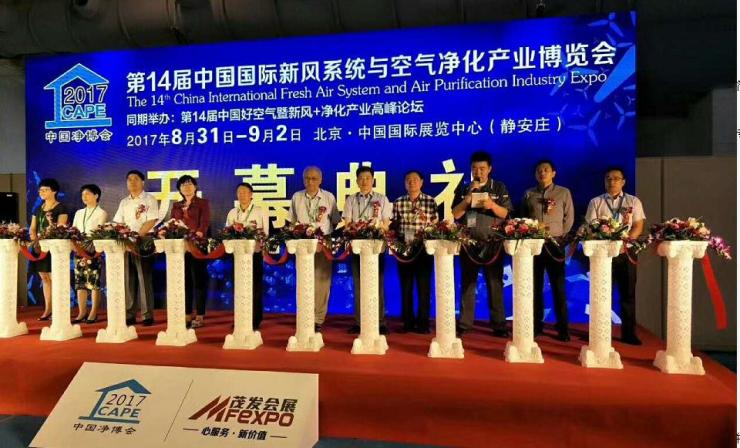继上次“2017第五届北京空气净化及新风系统展览会”后，洁琅新风作为湖北环保科技的高新技术企业和中国智能无管道新风系统的领航者，乘势而上，携吊顶机、柜机、壁挂机、筒机四大类别的新风机，再次惊艳亮相本次展会。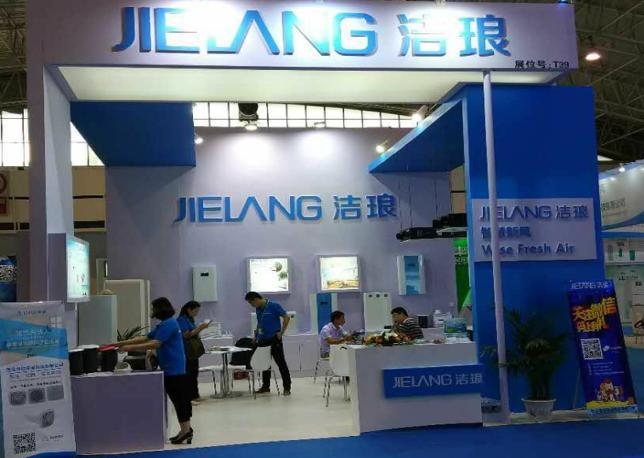湖北智能家居的新晋品牌——洁琅新风，由武汉维控环保科技有限公司研发并生产，维控环保成立于2009年，专注于空气净化、VOCS治理领域八载，为一家集科研、设计、制造和销售为一体的专业性环保科技企业。公司以环保产品设计及工程施工经验技术为核心，以技术创新指导产品开发并不断优化产品设计，针对环保产品尤其是空净产品的市场特点，能为用户提供个性化的产品解决方案。

洁琅新风本次参展的四类展品如下:

1)洁琅柜式系列新风机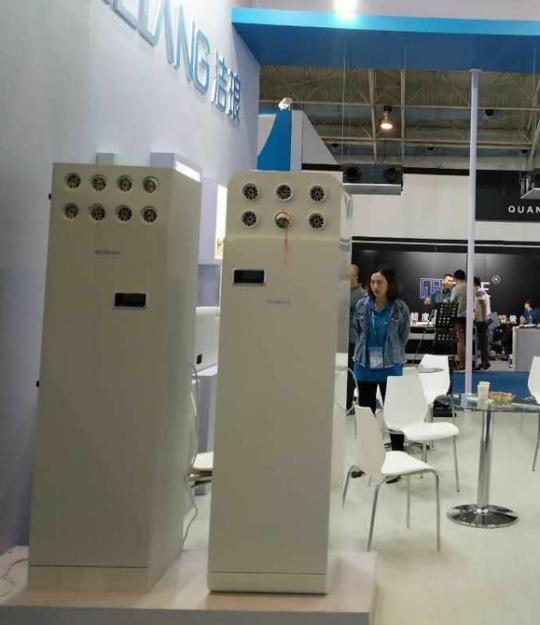洁琅柜机系列产品尤其适合安装在100平方~260平方的密闭空间，比如会议室、教室、医院或者移动营业厅等场地宽敞的公共场所。

产品优势：

高效净化：PM2.5净化效率高达99.99%

超低噪音：采用日本310V直流无刷电机，提高通风效率，运行最大噪音低于48分贝

节能换热：采用交叉逆流式显热交换器，换热率高达85%，

极致安全：具有维护开盖断电保护功能，产品自带防火装置

遥控/APP智能控制：室内空气质量实时显示，通过室内空气质量，自动调控设备

超大面积过滤网：超大的展开滤网面积，超长的滤网更换周期，使用更省心，维护更方便。

2)洁琅吊顶系列新风机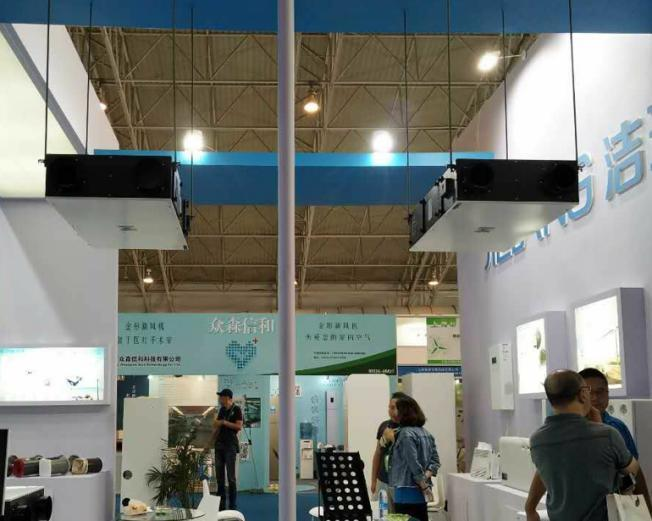洁琅吊顶机系列产品尤其适合安装在毛坯房，主要针对别墅、复式楼这样的豪宅式的大空间住宅人群。需要安装管道。必须在装修之前就做好相关准备。

产品优势：

高效净化：PM2.5净化效率高达99.99%

超低噪音：运行最大噪音低于39分贝

节能换热：采用全热交换器，换热率高达83%，节能减排

智能控制：室内空气质量实时显示，通过室内空气质量，自动调控设备

交换滤芯：全进口高效全热交换滤芯，有效调节新风温湿度，使用寿命长

360°送风：送风方式灵活，有顶送风、地送风和墙送风等多种形式，满足多种体感需求

3)洁琅壁挂系列新风机洁琅壁挂系列产品尤其适合安装在普通的30-100平方的三居室、二居室的客厅、儿童房等对空气质量要求高的独立空间。新房、存量房均适合，无需管道，安装方便、快捷。

产品优势：

高效净化：PM2.5净化效率高达99.99%

超低噪音：采用日本直流无刷电机，提高通风效率，运行最大噪音低于35分贝

节能换热：采用交叉逆流式显热交换器，换热率高达85%，

极致安全：具有维护开盖断电保护功能，产品自带防火装置

遥控/APP智能控制：室内空气质量实时显示，通过室内空气质量，自动调控设备

超大面积过滤网：超大的展开滤网面积，超长的滤网更换周期，使用更省心，维护更方便。

4)洁琅筒式系列新风机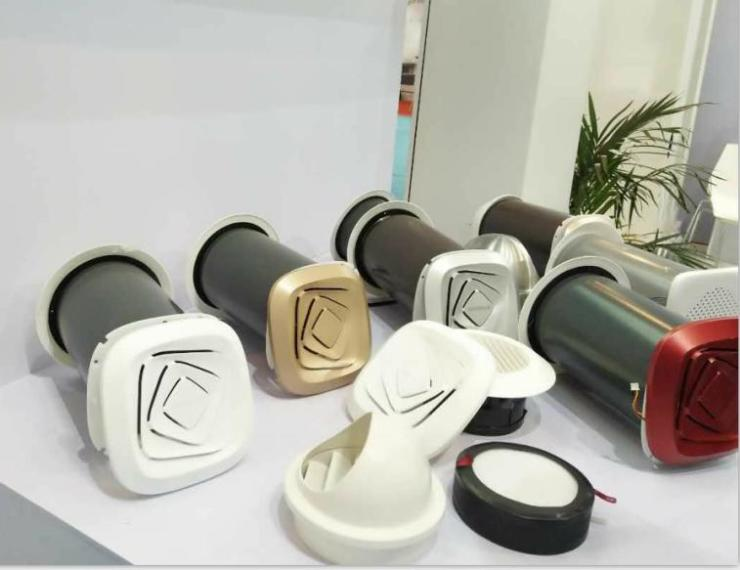尤其是本次重磅推出的筒式系列新风机，该款机器是洁琅新风的专利产品。小巧精致、无管道、安装方便快捷，且不破坏现有家居环境，不占空间，能与现有家居融为一体。此外，该款产品还具备噪音超低、保护居住私密性等独有的优点。在市场上琳琅满目的新风机器中独树一帜。其更先进的科技及独特的除霾除尘方式和传统新风系统相对庞大、笨重的外形有天壤之别。让参观者顿生好感。（此照片上为筒机模具，为的是更好的向参会展人群展示筒机的内部构造）

产品优势：

高效净化：PM2.5净化效率高达99.99%

超低噪音：采用独特造型风轮，提高通风效率，运营噪音低于32分贝

节能换热：采用单向显热交换器，换热率高达65%，节能减排

极致安全：整机使用韩国进口工程塑料制造，

智能控制：多种模式自由切换，完美匹配空间所需。

洁琅新风系统的工作原理为——从室外引进洁净新风，在气温极低的天气，回收大部分热量；在气温极高的天气，回收大部分冷量，在保持会议室等面积较大的空间的空气质量的同时，将夏季制冷、冬季采暖的能量损失，控制在最低水平，从而达到在净化空气的同时节能降耗的目的。

洁琅新风系统由武汉维控环保科技有限公司生产，公司为环保部门备案企业，以先进的高科技产品、可靠的产品质量、高效快捷的客户服务、诚实守信的经营理念，为二十一世纪人类生存环境的改善及高品质生活的提升而不懈努力。

2017~2018年，匠心独具的洁琅新风将乘势而上，推出更多适合中国家庭的新风产品，造福更多中国百姓！

无论你是有意愿为改善室内呼吸环境而奋斗的伙伴还是愿意在当地市场携手开拓洁琅新风市场的创业者，都请及时与洁琅新风联系！让我们并肩同行，共谋财富大业！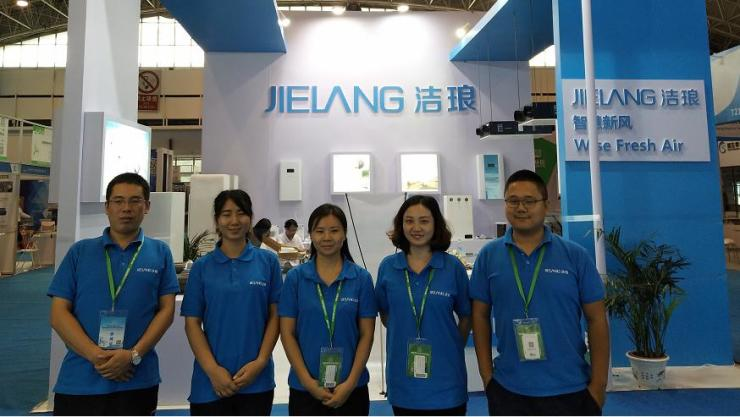`声明：本文由入驻焦点开放平台的作者撰写，除焦点官方账号外，观点仅代表作者本人，不代表焦点立场错误信息举报电话： 400-099-0099，邮箱：jubao@vip.sohu.com，或点此进行意见反馈，或点此进行举报投诉。`A B C D E F G H J K L M N P Q R S T W X Y Z
A - B - C - D - E
• A
• 鞍山
• 安庆
• 安阳
• 安顺
• 安康
• 澳门
• B
• 北京
• 保定
• 包头
• 巴彦淖尔
• 本溪
• 蚌埠
• 亳州
• 滨州
• 北海
• 百色
• 巴中
• 毕节
• 保山
• 宝鸡
• 白银
• 巴州
• C
• 承德
• 沧州
• 长治
• 赤峰
• 朝阳
• 长春
• 常州
• 滁州
• 池州
• 长沙
• 常德
• 郴州
• 潮州
• 崇左
• 重庆
• 成都
• 楚雄
• 昌都
• 慈溪
• 常熟
• D
• 大同
• 大连
• 丹东
• 大庆
• 东营
• 德州
• 东莞
• 德阳
• 达州
• 大理
• 德宏
• 定西
• 儋州
• 东平
• E
• 鄂尔多斯
• 鄂州
• 恩施
F - G - H - I - J
• F
• 抚顺
• 阜新
• 阜阳
• 福州
• 抚州
• 佛山
• 防城港
• G
• 赣州
• 广州
• 桂林
• 贵港
• 广元
• 广安
• 贵阳
• 固原
• H
• 邯郸
• 衡水
• 呼和浩特
• 呼伦贝尔
• 葫芦岛
• 哈尔滨
• 黑河
• 淮安
• 杭州
• 湖州
• 合肥
• 淮南
• 淮北
• 黄山
• 菏泽
• 鹤壁
• 黄石
• 黄冈
• 衡阳
• 怀化
• 惠州
• 河源
• 贺州
• 河池
• 海口
• 红河
• 汉中
• 海东
• 怀来
• I
• J
• 晋中
• 锦州
• 吉林
• 鸡西
• 佳木斯
• 嘉兴
• 金华
• 景德镇
• 九江
• 吉安
• 济南
• 济宁
• 焦作
• 荆门
• 荆州
• 江门
• 揭阳
• 金昌
• 酒泉
• 嘉峪关
K - L - M - N - P
• K
• 开封
• 昆明
• 昆山
• L
• 廊坊
• 临汾
• 辽阳
• 连云港
• 丽水
• 六安
• 龙岩
• 莱芜
• 临沂
• 聊城
• 洛阳
• 漯河
• 娄底
• 柳州
• 来宾
• 泸州
• 乐山
• 六盘水
• 丽江
• 临沧
• 拉萨
• 林芝
• 兰州
• 陇南
• M
• 牡丹江
• 马鞍山
• 茂名
• 梅州
• 绵阳
• 眉山
• N
• 南京
• 南通
• 宁波
• 南平
• 宁德
• 南昌
• 南阳
• 南宁
• 内江
• 南充
• P
• 盘锦
• 莆田
• 平顶山
• 濮阳
• 攀枝花
• 普洱
• 平凉
Q - R - S - T - W
• Q
• 秦皇岛
• 齐齐哈尔
• 衢州
• 泉州
• 青岛
• 清远
• 钦州
• 黔南
• 曲靖
• 庆阳
• R
• 日照
• 日喀则
• S
• 石家庄
• 沈阳
• 双鸭山
• 绥化
• 上海
• 苏州
• 宿迁
• 绍兴
• 宿州
• 三明
• 上饶
• 三门峡
• 商丘
• 十堰
• 随州
• 邵阳
• 韶关
• 深圳
• 汕头
• 汕尾
• 三亚
• 三沙
• 遂宁
• 山南
• 商洛
• 石嘴山
• T
• 天津
• 唐山
• 太原
• 通辽
• 铁岭
• 泰州
• 台州
• 铜陵
• 泰安
• 铜仁
• 铜川
• 天水
• 天门
• W
• 乌海
• 乌兰察布
• 无锡
• 温州
• 芜湖
• 潍坊
• 威海
• 武汉
• 梧州
• 渭南
• 武威
• 吴忠
• 乌鲁木齐
X - Y - Z
• X
• 邢台
• 徐州
• 宣城
• 厦门
• 新乡
• 许昌
• 信阳
• 襄阳
• 孝感
• 咸宁
• 湘潭
• 湘西
• 西双版纳
• 西安
• 咸阳
• 西宁
• 仙桃
• 西昌
• Y
• 运城
• 营口
• 盐城
• 扬州
• 鹰潭
• 宜春
• 烟台
• 宜昌
• 岳阳
• 益阳
• 永州
• 阳江
• 云浮
• 玉林
• 宜宾
• 雅安
• 玉溪
• 延安
• 榆林
• 银川
• Z
• 张家口
• 镇江
• 舟山
• 漳州
• 淄博
• 枣庄
• 郑州
• 周口
• 驻马店
• 株洲
• 张家界
• 珠海
• 湛江
• 肇庆
• 中山
• 自贡
• 资阳
• 遵义
• 昭通
• 张掖
• 中卫

1室1厅1厨1卫1阳台

1
2
3
4
5

0
1
2

1

1

0
1
2
3报名成功，资料已提交审核A B C D E F G H J K L M N P Q R S T W X Y Z
A - B - C - D - E
• A
• 鞍山
• 安庆
• 安阳
• 安顺
• 安康
• 澳门
• B
• 北京
• 保定
• 包头
• 巴彦淖尔
• 本溪
• 蚌埠
• 亳州
• 滨州
• 北海
• 百色
• 巴中
• 毕节
• 保山
• 宝鸡
• 白银
• 巴州
• C
• 承德
• 沧州
• 长治
• 赤峰
• 朝阳
• 长春
• 常州
• 滁州
• 池州
• 长沙
• 常德
• 郴州
• 潮州
• 崇左
• 重庆
• 成都
• 楚雄
• 昌都
• 慈溪
• 常熟
• D
• 大同
• 大连
• 丹东
• 大庆
• 东营
• 德州
• 东莞
• 德阳
• 达州
• 大理
• 德宏
• 定西
• 儋州
• 东平
• E
• 鄂尔多斯
• 鄂州
• 恩施
F - G - H - I - J
• F
• 抚顺
• 阜新
• 阜阳
• 福州
• 抚州
• 佛山
• 防城港
• G
• 赣州
• 广州
• 桂林
• 贵港
• 广元
• 广安
• 贵阳
• 固原
• H
• 邯郸
• 衡水
• 呼和浩特
• 呼伦贝尔
• 葫芦岛
• 哈尔滨
• 黑河
• 淮安
• 杭州
• 湖州
• 合肥
• 淮南
• 淮北
• 黄山
• 菏泽
• 鹤壁
• 黄石
• 黄冈
• 衡阳
• 怀化
• 惠州
• 河源
• 贺州
• 河池
• 海口
• 红河
• 汉中
• 海东
• 怀来
• I
• J
• 晋中
• 锦州
• 吉林
• 鸡西
• 佳木斯
• 嘉兴
• 金华
• 景德镇
• 九江
• 吉安
• 济南
• 济宁
• 焦作
• 荆门
• 荆州
• 江门
• 揭阳
• 金昌
• 酒泉
• 嘉峪关
K - L - M - N - P
• K
• 开封
• 昆明
• 昆山
• L
• 廊坊
• 临汾
• 辽阳
• 连云港
• 丽水
• 六安
• 龙岩
• 莱芜
• 临沂
• 聊城
• 洛阳
• 漯河
• 娄底
• 柳州
• 来宾
• 泸州
• 乐山
• 六盘水
• 丽江
• 临沧
• 拉萨
• 林芝
• 兰州
• 陇南
• M
• 牡丹江
• 马鞍山
• 茂名
• 梅州
• 绵阳
• 眉山
• N
• 南京
• 南通
• 宁波
• 南平
• 宁德
• 南昌
• 南阳
• 南宁
• 内江
• 南充
• P
• 盘锦
• 莆田
• 平顶山
• 濮阳
• 攀枝花
• 普洱
• 平凉
Q - R - S - T - W
• Q
• 秦皇岛
• 齐齐哈尔
• 衢州
• 泉州
• 青岛
• 清远
• 钦州
• 黔南
• 曲靖
• 庆阳
• R
• 日照
• 日喀则
• S
• 石家庄
• 沈阳
• 双鸭山
• 绥化
• 上海
• 苏州
• 宿迁
• 绍兴
• 宿州
• 三明
• 上饶
• 三门峡
• 商丘
• 十堰
• 随州
• 邵阳
• 韶关
• 深圳
• 汕头
• 汕尾
• 三亚
• 三沙
• 遂宁
• 山南
• 商洛
• 石嘴山
• T
• 天津
• 唐山
• 太原
• 通辽
• 铁岭
• 泰州
• 台州
• 铜陵
• 泰安
• 铜仁
• 铜川
• 天水
• 天门
• W
• 乌海
• 乌兰察布
• 无锡
• 温州
• 芜湖
• 潍坊
• 威海
• 武汉
• 梧州
• 渭南
• 武威
• 吴忠
• 乌鲁木齐
X - Y - Z
• X
• 邢台
• 徐州
• 宣城
• 厦门
• 新乡
• 许昌
• 信阳
• 襄阳
• 孝感
• 咸宁
• 湘潭
• 湘西
• 西双版纳
• 西安
• 咸阳
• 西宁
• 仙桃
• 西昌
• Y
• 运城
• 营口
• 盐城
• 扬州
• 鹰潭
• 宜春
• 烟台
• 宜昌
• 岳阳
• 益阳
• 永州
• 阳江
• 云浮
• 玉林
• 宜宾
• 雅安
• 玉溪
• 延安
• 榆林
• 银川
• Z
• 张家口
• 镇江
• 舟山
• 漳州
• 淄博
• 枣庄
• 郑州
• 周口
• 驻马店
• 株洲
• 张家界
• 珠海
• 湛江
• 肇庆
• 中山
• 自贡
• 资阳
• 遵义
• 昭通
• 张掖
• 中卫• 手机• 分享
• 设计
免费设计
• 计算器
装修计算器
• 入驻
合作入驻
• 联系
联系我们
• 置顶
返回顶部